Next: Classical Light Waves Up: Wave-Particle Duality Previous: Plane Waves

# Representation of Waves via Complex Functions

In mathematics, the symbol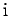is conventionally used to represent the square-root of minus one: i.e., one of the solutions of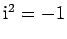. Now, a real number,(say), can take any value in a continuum of different values lying betweenand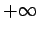. On the other hand, an imaginary number takes the general form, whereis a real number. It follows that the square of a real number is a positive real number, whereas the square of an imaginary number is a negative real number. In addition, a general complex number is written(36)

whereandare real numbers. In fact,is termed the real part of, andthe imaginary part of. This is written mathematically as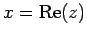and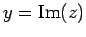. Finally, the complex conjugate ofis defined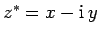.

Now, just as we can visualize a real number as a point on an infinite straight-line, we can visualize a complex number as a point in an infinite plane. The coordinates of the point in question are the real and imaginary parts of the number: i.e.,. This idea is illustrated in Fig. 3. The distance,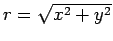, of the representative point from the origin is termed the modulus of the corresponding complex number,. This is written mathematically as. Incidentally, it follows that. The angle,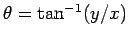, that the straight-line joining the representative point to the origin subtends with the real axis is termed the argument of the corresponding complex number,. This is written mathematically as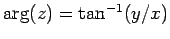. It follows from standard trigonometry that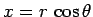, and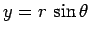. Hence,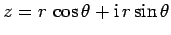.Complex numbers are often used to represent wavefunctions. All such representations depend ultimately on a fundamental mathematical identity, known as de Moivre's theorem, which takes the form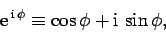(37)

where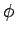is a real number. Incidentally, given that, whereis a general complex number,its modulus, and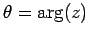its argument, it follows from de Moivre's theorem that any complex number,, can be written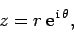(38)

whereandare real numbers.

Now, a one-dimensional wavefunction takes the general form(39)

whereis the wave amplitude,the wavenumber,the angular frequency, and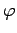the phase angle. Consider the complex wavefunction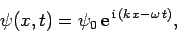(40)

where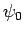is a complex constant. We can write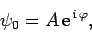(41)

whereis the modulus, andthe argument, of. Hence, we deduce that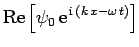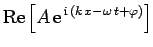(42)

Thus, it follows from de Moirve's theorem, and Eq. (39), that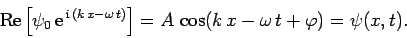(43)

In other words, a general one-dimensional real wavefunction, (39), can be represented as the real part of a complex wavefunction of the form (40). For ease of notation, the take the real part'' aspect of the above expression is usually omitted, and our general one-dimension wavefunction is simply written(44)

The main advantage of the complex representation, (44), over the more straightforward real representation, (39), is that the former enables us to combine the amplitude,, and the phase angle,, of the wavefunction into a single complex amplitude,. Finally, the three dimensional generalization of the above expression is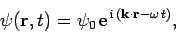(45)

where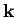is the wavevector.Next: Classical Light Waves Up: Wave-Particle Duality Previous: Plane Waves
Richard Fitzpatrick 2010-07-20